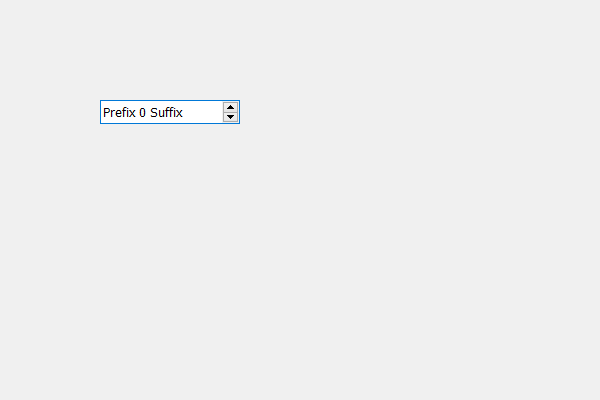GFG App
Open AppBrowser
Continue

# PyQt5 QSpinBox – Adjusting size according to the text

In this article we will see how we can adjust the size of the spin box according to the text in it. Somethings when we create a spin box and its value increases then the text size also increases therefore it is required to set the size of spin box such that it covers the whole text ago it didn’t take extra space as well. The text includes the prefix, postfix and the value with its length become maximum. In order to do this we use adjustSize method.

Syntax : spin_box.adjustSize() Argument : It takes no argument Action performed : It changes the size of the spin box according to the text

Below is the implementation

## Python3

 `# importing libraries` `from` `PyQt5.QtWidgets ``import` `*` `from` `PyQt5 ``import` `QtCore, QtGui` `from` `PyQt5.QtGui ``import` `*` `from` `PyQt5.QtCore ``import` `*` `import` `sys`     `class` `Window(QMainWindow):`   `    ``def` `__init__(``self``):` `        ``super``().__init__()`   `        ``# setting title` `        ``self``.setWindowTitle("Python ")`   `        ``# setting geometry` `        ``self``.setGeometry(``100``, ``100``, ``600``, ``400``)`   `        ``# calling method` `        ``self``.UiComponents()`   `        ``# showing all the widgets` `        ``self``.show()`   `    ``# method for widgets` `    ``def` `UiComponents(``self``):` `        ``# creating spin box` `        ``self``.spin ``=` `QSpinBox(``self``)`   `        ``# setting geometry to spin box` `        ``self``.spin.setGeometry(``100``, ``100``, ``250``, ``40``)`   `        ``# setting prefix to spin` `        ``self``.spin.setPrefix("Prefix ")`   `        ``# setting suffix to spin` `        ``self``.spin.setSuffix(" Suffix")`   `        ``# setting range to the spin box` `        ``self``.spin.setRange(``0``, ``999999``)`   `        ``# adjusting the size of the spin box` `        ``self``.spin.adjustSize()`     `# create pyqt5 app` `App ``=` `QApplication(sys.argv)`   `# create the instance of our Window` `window ``=` `Window()`   `# start the app` `sys.exit(App.``exec``())`

Output :My Personal Notes arrow_drop_up